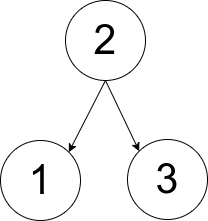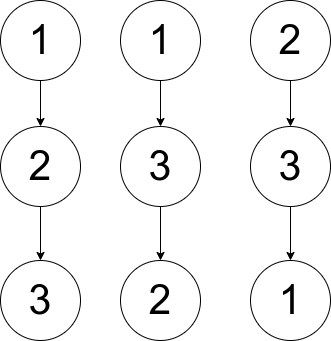# GeetCode Hub

You are given an array `pairs`, where `pairs[i] = [xi, yi]`, and:

• There are no duplicates.
• `xi < yi`

Let `ways` be the number of rooted trees that satisfy the following conditions:

• The tree consists of nodes whose values appeared in `pairs`.
• A pair `[xi, yi]` exists in `pairs` if and only if `xi` is an ancestor of `yi` or `yi` is an ancestor of `xi`.
• Note: the tree does not have to be a binary tree.

Two ways are considered to be different if there is at least one node that has different parents in both ways.

Return:

• `0` if `ways == 0`
• `1` if `ways == 1`
• `2` if `ways > 1`

A rooted tree is a tree that has a single root node, and all edges are oriented to be outgoing from the root.

An ancestor of a node is any node on the path from the root to that node (excluding the node itself). The root has no ancestors.

Example 1:```Input: pairs = [[1,2],[2,3]]
Output: 1
Explanation: There is exactly one valid rooted tree, which is shown in the above figure.
```

Example 2:```Input: pairs = [[1,2],[2,3],[1,3]]
Output: 2
Explanation: There are multiple valid rooted trees. Three of them are shown in the above figures.
```

Example 3:

```Input: pairs = [[1,2],[2,3],[2,4],[1,5]]
Output: 0
Explanation: There are no valid rooted trees.```

Constraints:

• `1 <= pairs.length <= 105`
• `1 <= xi < yi <= 500`
• The elements in `pairs` are unique.

class Solution { public int checkWays(int[][] pairs) { } }